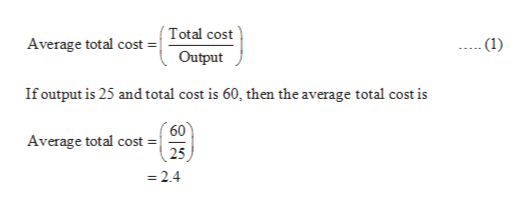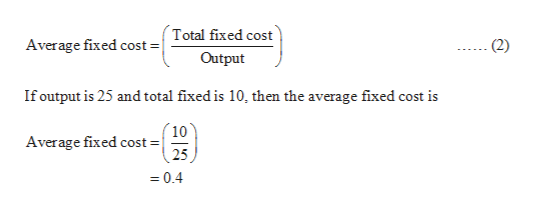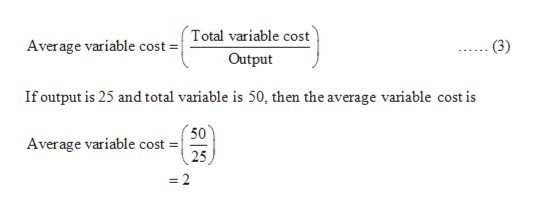# The cost structure of a manufacturer of microchips is described in the table in the next column. The firm’s fixed costs equal to \$10 per day. Calculate the average variable cost, average fixed cost, and average total cost at each output level. Output (microchips per day)Total Cost of Output (\$ thousands)0102560509575150100220125325150465

Question
1 views

The cost structure of a manufacturer of microchips is described in the table in the next column. The firm’s fixed costs equal to \$10 per day. Calculate the average variable cost, average fixed cost, and average total cost at each output level.

 Output (microchips per day) Total Cost of Output (\$ thousands) 0 10 25 60 50 95 75 150 100 220 125 325 150 465

check_circle

Step 1

Average total cost:

Average total cost can be calculated by using the following formula:help_outlineImage TranscriptioncloseTotal cost (1) Average total cost Output If output is 25 and total cost is 60, then the average total cost is 60 Average total cost =| 25 2.4 fullscreen
Step 2

Average fixed cost:

Average fixed cost can be calculated by using the following formula:help_outlineImage TranscriptioncloseTotal fixed cost Average fixed cost = (2) Output If output is 25 and total fixed is 10, then the average fixed cost is ´10 Average fixed cost = 25 = 0.4 fullscreen
Step 3

Average variable cost:

Average variable cost can be calcula...help_outlineImage TranscriptioncloseTotal variable cost Average variable cost =| (3) Output If output is 25 and total variable is 50, then the average variable cost is 50 Average variable cost 25 2 fullscreen

### Want to see the full answer?

See Solution

#### Want to see this answer and more?

Solutions are written by subject experts who are available 24/7. Questions are typically answered within 1 hour.*

See Solution
*Response times may vary by subject and question.
Tagged in